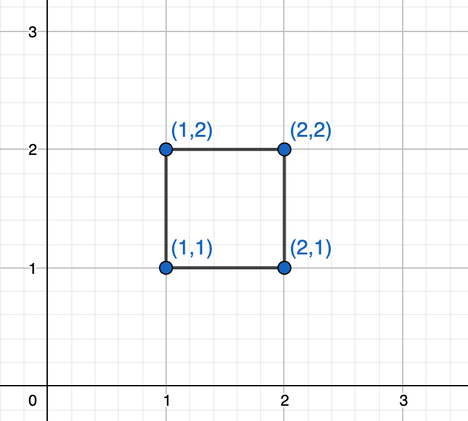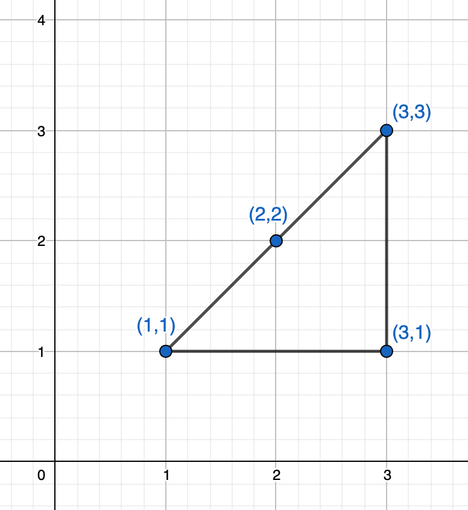# Rectangle or NotHasinur_Replay of CodeSpark 2021
Limits 1s, 512 MB

Let’s imagine a quadrant lies on a 2D coordinate system’s first quadrant. you will be given the coordinates of each vertex. Your task is to determine if it is an axis parallel, non-degenerate rectangle or not.

Axis parallel rectangle is a geometric shape which has sides parallel to axes of the coordinate system.

A rectangle is called non-degenerate if it is not deformed. Hence, there are no three vertices lying on the same straight line and the rectangle has a positive area. Read sample case explanation for better understanding.

## Input

The first line of the input will contain a single integer $T$ $(1 \leq T \leq 10^5)$which denotes the number of test cases. Then, there will be $T$ test cases.

Each of the next $T$ lines will contain eight positive integers separated by spaces, representing the coordinates of the vertices. To clarify things a little bit, let there be four vertices of the quadrant $(x_1, y_1)$, $(x_2, y_2)$, $(x_3, y_3)$, $(x_4, y_4)$ where $1 \leq x_i, y_i \leq 10^5$. Then the input will contain the coordinates in a single line like below:

$x_1$ $y_1$ $x_2$ $y_2$ $x_3$ $y_3$ $x_4$ $y_4$

Note that, the vertices will not be given in any particular order.

## Output

For each test case, print “yes” without quotations if the given quadrant is an axis parallel, non-degenerate quadrilateral rectangle in a line. Print “no” otherwise. You can print each letter in any case. For example, “YeS”, ”YES”, “yES”, “no”, ”NO” etc. are also acceptable.

## Sample

InputOutput
5
1 1 1 2 2 2 2 1
1 2 2 1 1 1 2 2
1 1 1 1 1 1 1 1
1 1 2 2 1 2 2 3
1 1 2 2 3 3 3 1

yes
yes
no
no
no


Explanation of the first test case:The sides are parallel to the axes and there are no three vertices lying on the same straight line. The area is positive.

This is an axis parallel, non-degenerate rectangle.

Explanation of the fifth test case:There are three vertices lying on the same straight line. So, this is not a non-degenerated rectangle.

### Submit